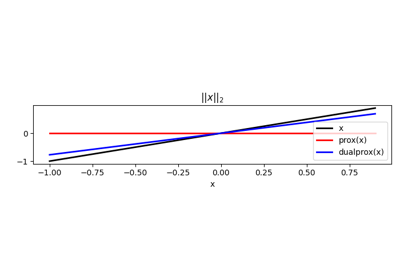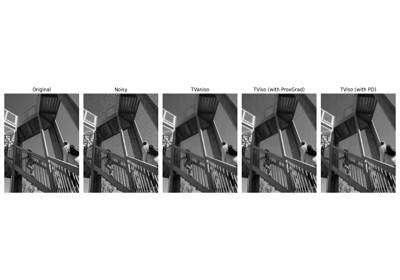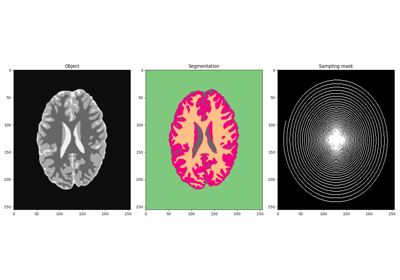# pyproximal.L21#

class pyproximal.L21(ndim, sigma=1.0)[source]#

$$L_{2,1}$$ proximal operator.

Proximal operator for $$L_{2,1}$$ matrix norm.

Parameters
ndimint

Number of dimensions $$N_{dim}$$. Used to reshape the input array in a matrix of size $$N_{dim} \times N'_{x}$$ where $$N'_x = \frac{N_x}{N_{dim}}$$. Note that the input vector x should be created by stacking vectors from different dimensions.

sigmafloat, optional

Multiplicative coefficient of $$L_{2,1}$$ norm

Notes

Given the $$L_{2,1}$$ norm of a matrix of size $$N_{dim} \times N'_x$$ defined as:

$\sigma \|\mathbf{X}\|_{2,1} = \sigma \sum_{j=0}^{N'_x} \|\mathbf{x}_j\|_2 = \sigma \sum_{j=0}^{N'_x} \sqrt{\sum_{i=0}^{N_{dim}}} |x_{ij}|^2$

the proximal operator is:

$\prox_{\tau \sigma \|\cdot\|_{2,1}}(\mathbf{x}_j) = \left(1 - \frac{\sigma \tau}{max\{||\mathbf{x}_j||_2, \sigma \tau \}}\right) \mathbf{x}_j \quad \forall j$

Similar to the Euclidean norm, the dual operator is defined as:

$\prox^*_{\tau \sigma \||\cdot\|_{2,1}}(\mathbf{x}_j) = \frac{\sigma \mathbf{x}_j}{\max\{||\mathbf{x}_j||_2, \sigma\}} \quad \forall j$

Finally, we note that the $$L_{2,1}$$ norm is a separable function on each column on the matrix $$\mathbf{X}$$. Taking advantage of the property of proximal operator of separable function , its proximal and dual proximal operators can be interpreted as a series of pyproximal.proximal.Euclidean operators on each column of the matrix $$\mathbf{X}$$.

1

N., Parikh, “Proximal Algorithms”, Foundations and Trends in Optimization. 2013.

Methods

 __init__(ndim[, sigma]) affine_addition(v) Affine addition chain(g) Chain grad(x) Compute gradient postcomposition(sigma) Postcomposition precomposition(a, b) Precomposition prox(**kwargs) proxdual(**kwargs)

## Examples using pyproximal.L21#Norms

Norms